# Chemical equation

## Octet rule

As mentioned at some of the previous chapters, the electron configuration of the noble gases is very stable. Besides helium, all other noble gases feature eight valence electrons (the outermost s-orbital is filled with 2 electrons and the p-orbital is filled with 6 electrons). Atoms with a different number of valence electrons tend to form a similar configuration by combining in such a way that each have eight electrons in the outermost shell. This tendency to form a valence shell containing eight electrons is especially given for the main group elements (group 1, 2 and 13-17) and is called the octet rule. One prominent exception of this rule is hydrogen, because the noble gas configuration of it's period is given by just two valence electrons accordant to the noble gas helium. The tendency to reach the noble gas configuration of helium is called the duet rule. Lithium and beryllium are two more elements following those rule.
Chemical reactions occur between atoms in order to archive a complete octet respectively duet of valence electrons. The main types of chemical bonds are ionic and covalent bonds meaning atoms loose, gain or share valence electrons.

## Lewis structure

The bonding between atoms of a molecule is often drawn in diagrams. The American chemist Gilbert Newton Lewis introduced in 1916 an often used diagram type showing the valence electrons and the bonding of atoms in a molecule. He used the chemical symbol of the chemical elements to show each atom and it's position in the structure of molecules. Electron pairs being shared by forming a covalent bond are represented by a line between the participating atoms. The valence electrons not being involved in a valence bond are represented as pairs of dots placed next to the atom: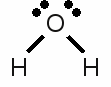Lewis structure of a water molecule:
Hydrogen has one valence electron, oxygen has six valence electrons. Two of the oxygens valence electrons are forming covalent bondings with hydrogen atoms, represented by a line between the atoms. The two pairs of electrons not being involved in a chemical bond are drawn as pairs of dots next to the symbol of the oxygen atom. In the drawn water molecule the oxygen atom attains eight valence electrons: Two lone pairs of electrons and two pairs of electrons shared with the hydrogen atoms. Each hydrogen atom attains two electrons by forming a covalent bond. The conditions of the octet respectively the duet rule are complied with.Lewis structure of an oxygen molecule:
The double bond between the two oxygen atoms is represented by the two lines between the chemical symbols and the lone pairs are drawn as pairs of dots. Each oxygen atom attains eight valence electrons (two lone pairs and two pairs of electrons being shared between the atoms).

The structures drawn above are called Lewis structures, also known as Lewis dot diagrams, electron dot diagrams, and electron dot structures.

## Ion

Besides sharing electrons by forming a covalent bond, atoms can also loose electrons to "clear" the outermost shell respectively gain electrons to "fill" their valence shell. One example for an ionic bond like this is sodium chloride. The sodium atom transfers it's single valence electron permanently to a chlorine atom resulting in a positively charged sodium ion and a negatively charged chlorine atom. Positively charged atoms are called cations, negatively charged ions are called anions.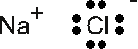Ionic bond of sodium chlorine:
One single chlorine atom has seven valence electrons. When gaining one more electron from the sodium atom it is forming a negatively charged chlorine anion. The sodium atom has lost its outermost electron forming a positively charged sodium cation.

Ions can also consist out of a mixture of covalent bond and ionic bond. An example is the carbonate ion:Two times negatively charged carbonate ion:
All participating atoms of this combined covalent and ionic bond feature eight valence electrons.

## Chemical equation

A chemical reaction is graphically represented by a chemical equation. The starting substances or reactants are written on the left hand side and the substances formed - the product(s) - at the right hand side separated by one ore two arrows. The arrows indicate the direction of the reaction. If hydrogen is burned in an oxygen containing atmosphere, water is produced by the chemical reaction: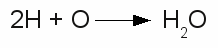The arrow is pointing from the left (2H + O) to the right (H2O). We will see some later that water also can be cracked into hydrogen and oxygen:Once more the reactant is written on the left side (H2O) and the products are written on the right side (2H + O). We will see some later that sometimes the conditions allow a reaction in both directions which is symbolized by two arrows: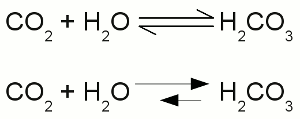Chemical reaction in both directions:
The upper drawing is used to denote the chemical equilibrium, meaning the concentration of reactants and products doesn't change over time.
The lower part of the drawing indicates that the equilibrium is not reached (yet). The rate of the reverse reaction (small arrow pointing from the right to the left) is lower than those of the forward chemical process (arrow pointing from the left to the right).

The reactants or products are separated by plus signs and the participating elements respectively substances are drawn by their chemical symbols respectively their empirical formula or molecular formula. The empirical formula of a chemical compound is the simplest whole number ratio of atoms of each element present in a compound. It is used as standard for most ionic compounds such as MgCl2 (magnesium chloride). The substring indicates the ratio of elements. MgCl2 indicates that magnesium chloride consists of magnesium and chlorine in a ratio of 1:2. This ratio can be detected by elemental analysis. The molecular formula or chemical formula expresses information about the number of atoms of each element found in each discrete molecule of a chemical compound. Like at the empirical formula, the subscript after the chemical symbol indicates that the molecule consists of more than one atom of the particular element. A simple example is the molecular formula of water molecules H2O. A more complex molecule is given by the formula C6H8O7 (citric acid).
The coefficients next to the chemical symbols or formulae indicates the absolute values of the stoichiometric numbers. A stoichiometric number gives the number of molecules respectively atoms participating in the reaction and denotes the relation among quantities of reactants and products. Typically the smallest whole numbers possible are used. When considering that hydrogen and oxygen gas is formed by hydrogen respectively oxygen molecules instead of single atoms, the chemical equation between hydrogen and oxygen is given by: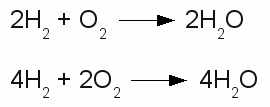Stoichiometric numbers:
The reaction between hydrogen and oxygen yields water. The reaction stoichiometry of hydrogen, oxygen and water molecules is 2:1:2 which is the same as 4:2:4. The upper version of the chemical equation is using the smallest whole numbers possible.
The equation would be read as: "Two H two plus O two yields H two O.".

The quantity of each element doesn't change in a chemical reaction, because of the conservation of mass. Thus the same number of atoms of each element must be at the left as well as at the right side of the equation. At the example above the number of hydrogen atoms at the left is 4 ( = 2 * H * 2) and the number of oxygen atoms is 2 ( = 1 * O * 2). At the right side we can count 4 hydrogen atoms ( = 2 * H *2O) and 2 oxygen atoms ( = 2 * H2O), too. The lower equation leads to 8 hydrogen atoms ( = 4 * H * 2 ) respectively 4 oxygen atoms ( = 2 * O * 2 ) at both sides.